2. 链式存储结构的线性表## 2.0. 线性表的链式存储结构

1.0 什么是线性表？ 中我们介绍了一种“用曲线连接”的线性表，“曲线”是一种形象化的语言，实际上并不会存在所谓“曲线”的这种东西。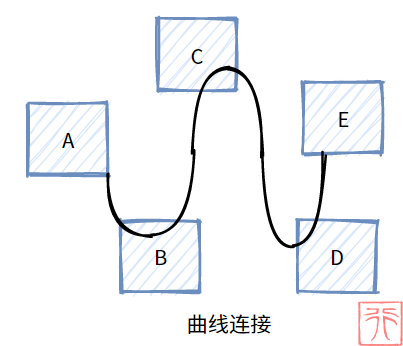1.1. 线性表的顺序存储方式中介绍顺序存储结构时举了一个例子：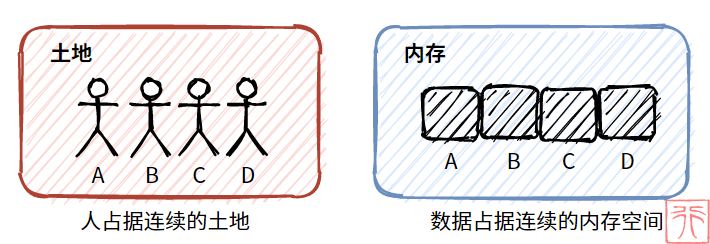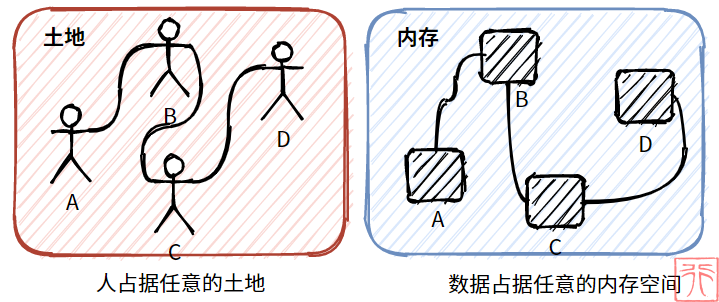• 直线 VS 曲线
• 整齐划一 VS 杂乱无章
• 连续内存空间 VS 任意内存空间
• 顺序存储 VS 链式存储

1.1. 线性表的顺序存储方式中提到过，线性表的特点之一是元素之间有顺序。顺序存储结构靠连续的内存空间来实现这种“顺序”，但链式存储结构的元素是“任意的”，如何实现“顺序”呢？

“任意”和“顺序”乍一看很像反义词，但并不矛盾。小孩子们为了不让队伍中断便“手拉手”，我们要实现“顺序”，就靠那根“像链条一样的曲线”来把元素链接起来，这样就有了顺序。

1.1. 线性表的顺序存储方式中提到过“顺序存储结构是使用一段连续的内存单元分别存储线性表中的数据的数据结构”。我们照猫画虎，就可以得到“链式存储结构是使用一组任意的、不连续的内存单元来分别存储线性表中的数据的数据结构”的结论。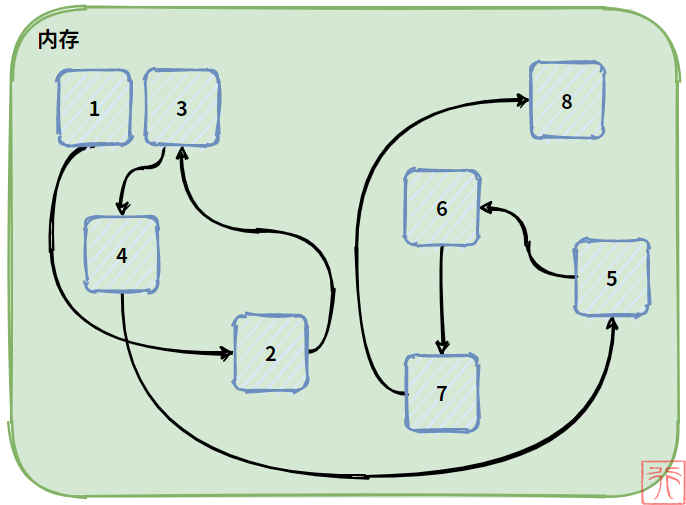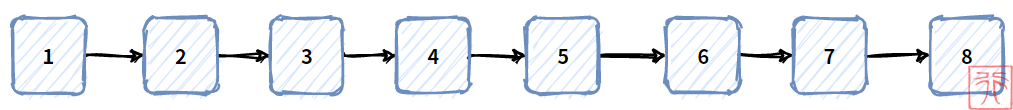1. 用一组任意的存储单元来存储线性表的数据元素，这组存储单元可以是连续的（1和3），也可以是不连续的；
2. 这组任意的存储单元用“一根链子”串起来，所以虽然在内存上是乱序的，但是在逻辑上却仍然是线性的；
3. 单链表的方向是单向的，只能从前往后，不能从后往前；

## 2.1. 单链表的实现思路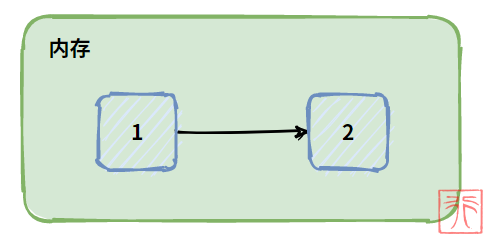C 语言刚好有这个机制——指针。如果你对指针还不清楚，可以移步到这篇文章——如何掌握 C 语言的一大利器——指针？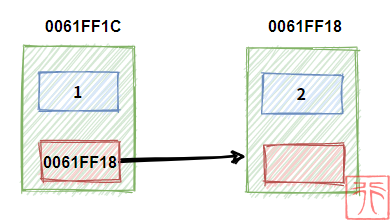• 用来存数据的变量叫做数据域
• 用来存“直接后继元素的地址”的 指针 叫做指针域
• 数据域和指针域构成的数据元素叫做 结点 (node)

• 用来存数据的数据域——`data`
• 用来存直接后继结点的的地址的指针域——`next`

## 2.2. 结点的具体实现

``````//结点
typedef struct Node {
int data; //数据域：存储数据
struct Node *next; //指针域：存储直接后继结点的地址
} Node;``````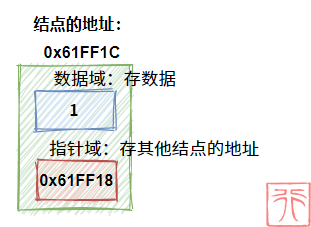## 2.3. 单链表的结构

### 2.3.0. 头指针

（为了方便理解链表的结构，我会给出每个结点的地址以及指针域的值）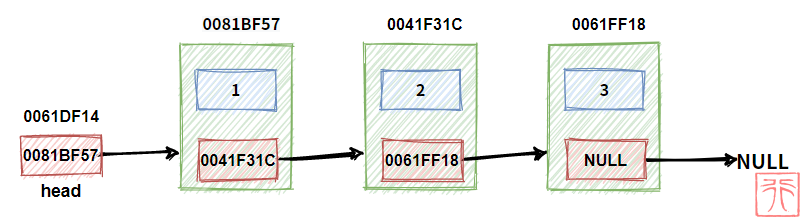### 2.3.1. 头结点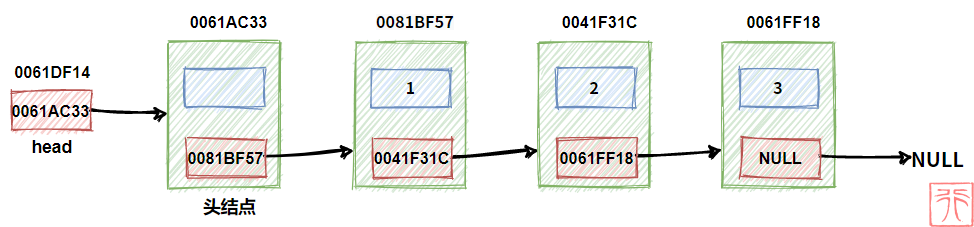### 2.3.2. 有无头结点的单链表

• 带头结点的单链表
• 不带头结点的单链表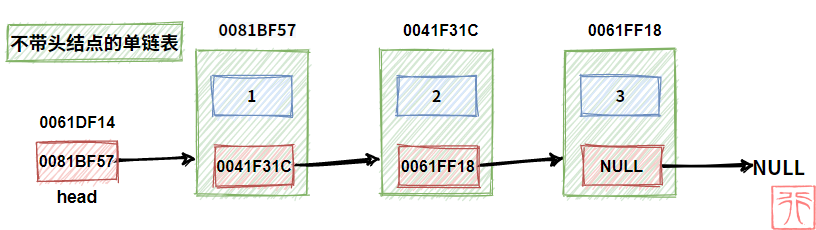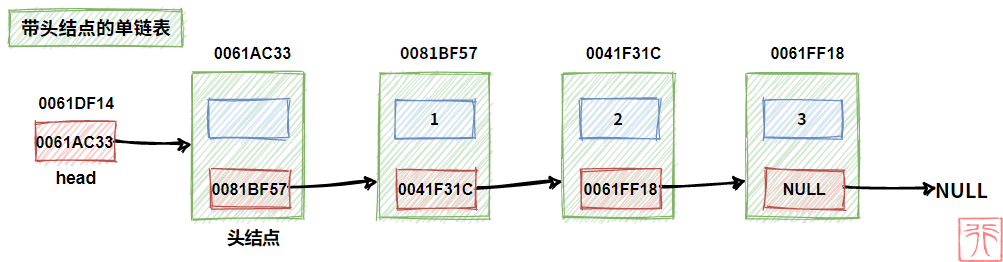• 头指针很重要，没它不行
• 虽然头结点可以方便我们的一些操作，但是有没有都行
• 无论带头结点与否，头指针都指向链表的第一个结点

（后面的操作我们将讨论不带头结点和带头结点两种情况）

## 2.4. 空链表

【不带头结点】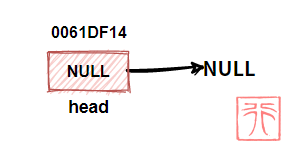【带头结点】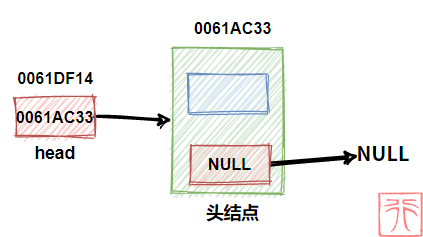（后面的图示将不再给出内存地址）

## 2.5. 创造结点

``````/**
* 创造结点，返回指向该结点的指针
* elem : 结点的数据域的值
* return : 指向该结点的指针（该结点的地址）
*/
Node *create_node(int elem)
{
Node *node = (Node *) malloc(sizeof(Node));
node->data = elem;
node->next = NULL;
return node;
}``````

## 2.6. 初始化链表

【不带头结点】

``Node *head = NULL;``

【带头结点】

1. 链表的头指针

2. 指向【指向结点的指针】的指针

3. 函数参数的值传递和地址传递

1. 头指针是链表一定要有的，找到头指针才能找到整个链表，否则整个链表就消失在“茫茫内存”之中了。所以无论进行何种操作，头指针一定要像我们攥紧绳子头一样被“攥”在我们手中。
2. 指针中保存了别人的地址，但它也有自己地址。如果一个指针中保存了别的指针的地址，该指针就是“指向指针的指针”。因为头指针是指向链表第一个结点的指针，所以我们找到头指针也就找到了整个链表（这句话啰嗦太多遍了）。而为了能找到头指针，我们就需要知道头指针的地址，也即将头指针的地址保存下来，换句话说，用一个指针来指向头指针
3. 函数的值传递改变的是形参（实参的一份拷贝），影响不了实参。所以在一些情况下，我们需要传给函数的是地址，函数使用指针来直接操作该指针指向的内存。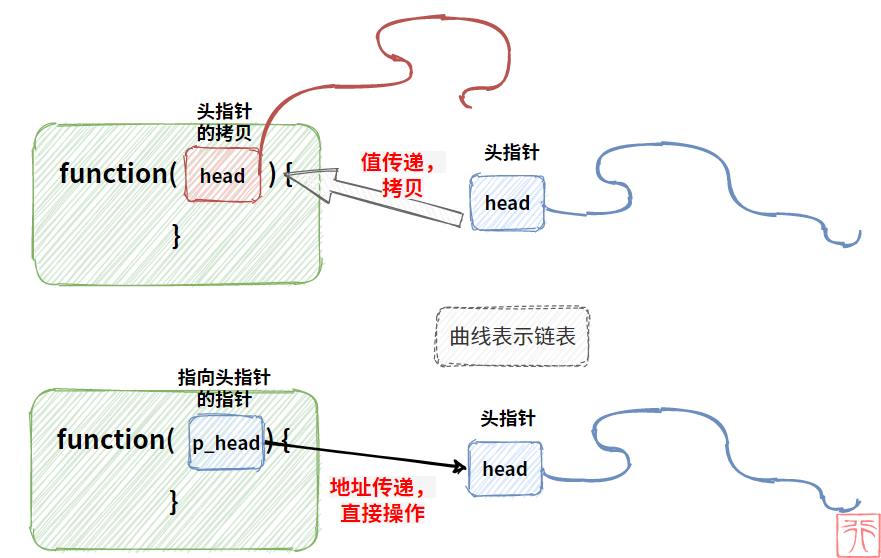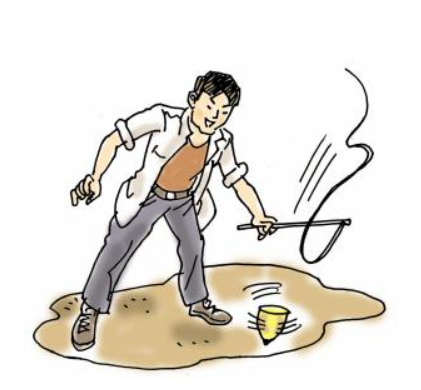``````/**
* 初始化链表
*/
{
//创建头结点
Node *node = (Node *) malloc(sizeof(Node));
node->next = NULL;
//头指针指向头结点
}``````

## 2.7. 遍历操作

【不带头结点】

``````int get_length(Node *head)
{
int length = 0;
while (p != NULL) {
length++;
p = p->next;
}
return length;
}``````

【带头结点】

``````int get_length(Node *head)
{
int length = 0;
while (p != NULL) {
length++;
p = p->next;
}
return length;
}``````

## 2.7. 插入操作

### 2.7.0. 基本思想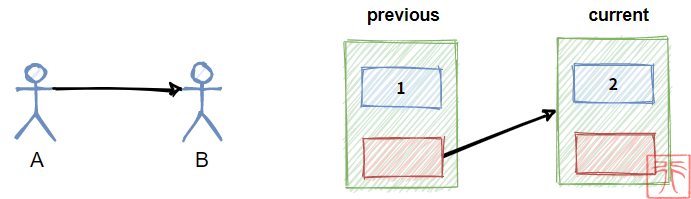C 拉上 B 的手 => A 松开 B 的手（虚线表示松开） => A 拉上 C 的手

A 松开 B 的手 => A 拉上 C 的手 => C 拉上 B 的手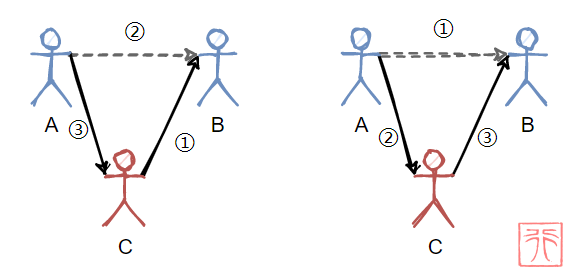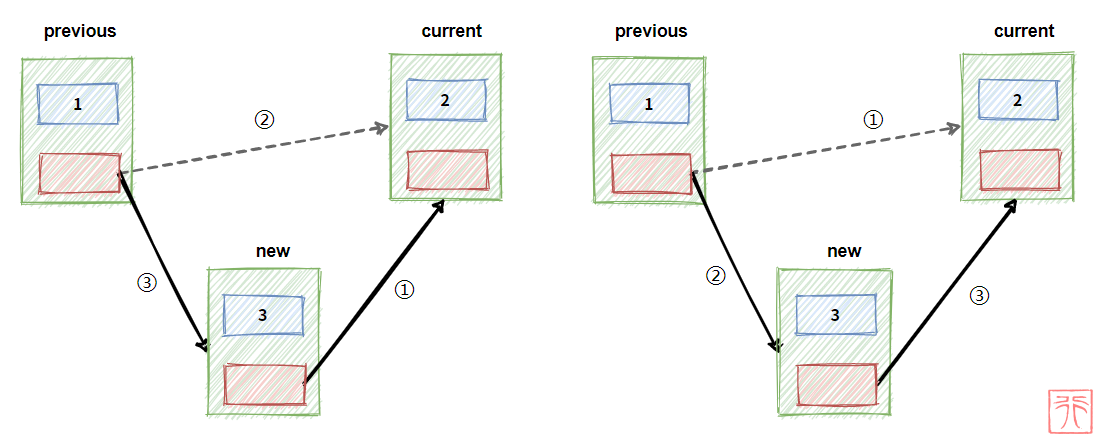``````new->next = current;
previous->next = new;``````

``````previous->next = new;
new->next = current;``````

``current = previous->next;``

``````new->next = previous->next;
previous->next = new;``````

``````// 错误代码
previous->next = new;
new->next = previous->next;``````

【角度一】

【角度二】

### 2.8.2. 指定位置插入

【不带头结点】

1. 在第一个元素前插入
2. 在其他元素前插入
``````/**
* 指定插入位置
* position: 指定位置 (1 <= position <= length + 1)
* elem: 新结点的数据
*/
void insert(Node **p_head, int position, int elem)
{
Node *new = create_node(elem);
Node *previous = NULL;
if (position < 1 || position > length + 1) {
printf("插入位置不合法\n");
return;
}
for (int i = 0; current != NULL && i < position - 1; i++) {
previous = current;
current = current->next;
}
new->next = current;
if (previous == NULL)
else
previous->next = new;
}``````

【带头结点】

``````/**
* 指定插入位置
* position: 指定位置 (1 <= position <= length + 1)
* elem: 新结点的数据
*/
void insert(Node **p_head, int position, int elem)
{
Node *new = create_node(elem);
Node *current = previous->next;
if (position < 1 || position > length + 1) {
printf("插入位置不合法\n");
return;
}
for (int i = 0; current != NULL && i < position - 1; i++) {
previous = current;
current = current->next;
}
new->next = current;
previous->next = new;
}``````

### 2.7.2. 头插法

【不带头结点】

``````/**
* 头插法：新插入的节点始终被头指针所指向
* elem: 新结点的数据
*/
{
Node *new = create_node(elem);
}``````

【带头结点】

``````/**
* 头插法，新结点为头结点的直接后继
* elem: 新结点的数据
*/
{
Node *new = create_node(elem);
}``````

### 2.7.3. 尾插法

【不带头结点】

``````/**
* 尾插法：新插入的结点始终在链表尾
* elem: 新结点的数据
*/
{
Node *new = create_node(elem);
while (p->next != NULL)   //从头遍历至链表尾
p = p->next;
p->next = new;
}``````

【带头结点】

``````/**
* 尾插法：新插入的结点始终在链表尾
* elem: 新结点的数据
*/
{
Node *new = create_node(elem);
while (p->next != NULL)
p = p->next;
p->next = new;
}``````

## 2.8. 删除操作

### 2.8.0. 基本思想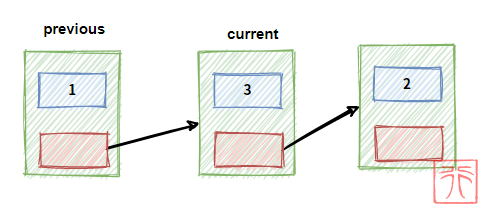`previous``current` 为指向结点的指针，现在我们要删除结点 `current`，过程如下：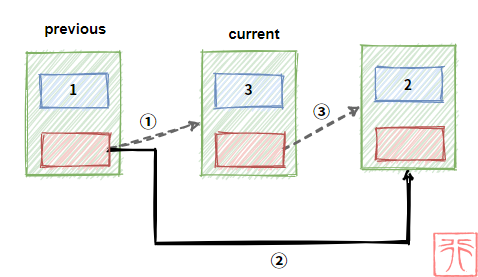``````previous->next = current->next;
free(current);``````

`free()` 操作将要删除的结点给释放掉。

`current` 指针不是必需的，没有它也可以，代码写成这样：

``previous->next = previous->next->next;``

### 2.8.2. 指定位置删除

【不带头结点】

``````/**
* 删除指定位置的结点
* position: 指定位置 (1 <= position <= length + 1)
* elem: 使用该指针指向的变量接收删除的值
*/
void delete(Node **p_head, int position, int *elem)
{
Node *previous = NULL;
if (length == 0) {
printf("空链表\n");
return;
}
if (position < 1 || position > length) {
printf("删除位置不合法\n");
return;
}
for (int i = 0; current->next != NULL && i < position - 1; i++) {
previous = current;
current = current->next;
}
*elem = current->data;
if (previous == NULL)
else
previous->next = current->next;
free(current);
}``````

【带头结点】

``````/**
* 删除指定位置的结点
* position: 指定位置 (1 <= position <= length + 1)
* elem: 使用该指针指向的变量接收删除的值
*/
void delete(Node **p_head, int position, int *elem)
{
Node *current = previous->next;
if (length == 0) {
printf("空链表\n");
return;
}
if (position < 1 || position > length) {
printf("删除位置不合法\n");
return;
}
for (int i = 0; current->next != NULL && i < position - 1; i++) {
previous = current;
current = current->next;
}
*elem = current->data;
previous->next = current->next;
free(current);
}``````

## 2.10. 单链表的优缺点

• 插入和删除某个元素时，不必像顺序表那样移动大量元素。
• 链表的长度不像顺序表那样是固定的，需要的时候就创建，不需要了就删除，极其方便。

• 单链表的查找和修改需要遍历链表，如果要查找的元素刚好是链表的最后一个，则需要遍历整个单链表，不像顺序表那样可以直接存取。22
Lv1
……
16

6

3